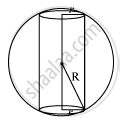# Show that the Height of the Cylinder of Maximum Volume, Which Can Be Inscribed in a Sphere of Radiu - Mathematics and Statistics

Sum

Show that the height of the cylinder of maximum volume, which can be inscribed in a sphere of radius R is (2R)/sqrt3.  Also find the maximum volume.

#### Solution

Given, radius of the sphere is R.

Let r and h be the radius and the height of the inscribed cylinder respectively.From the given figure, we have h=2sqrt(R^2-r^2)

The volume (V) of the cylinder is given by,

V=pir^2h=2pir^2sqrt(R^2-r^2)

therefore (dV)/(dr)=4pirsqrt(R^2-r^2)+(2pir^2(-2r))/(2sqrt(R^2-r^2))

=(4pirR^2-6pir^3)/sqrt(R^2-r^2)

for maxima or minima, (dV)/(dr) =0

(4pirR^2-6pir^3)=0

r^2=(2R^2)/3

Now ,(d^2V)/(dr^2)=(sqrt(R^2-r^2)(4piR^2-18pir^2)-(4piR^2-6pir^3)(-2r)/(2sqrt(R^2-r^2)))/(R^2-r^2)

=((R^2-r^2)(4piR^2-18pir^2)-r(4piR^2-6pir^3))/(R^2-r^2)^(3/2)

=(4piR^4-22pir^2R^2+12pir^4+4pir^2R^2)/(R^2-r^2)^(3/2)

Now, it can be observed that when r^2=(2RR62)/3,(d^2V)/(dr^2)<0

∴The volume is the maximum when r^2=(2R^2)/3

Maximum volume = V = pih((4R^2 - h^2)/4)
h = 2R/sqrt3

V_(max) = pi xx 2R/sqrt3 ((4R^2 - 4R^2/3)/4)

 = (2piR)/sqrt3 . (2R^2)/3 = (4piR^3)/(3sqrt3) cubic units

Hence, the volume of the cylinder is the maximum when the height of the cylinder is (2R)/3

Concept: Maxima and Minima
Is there an error in this question or solution?

#### APPEARS IN

NCERT Class 12 Maths
Chapter 6 Application of Derivatives
Exercise 6.6 | Q 17 | Page 243